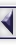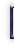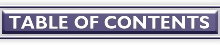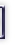## Some issues on floating-point precision under Linux

#### By Alan Ward

Abstract

In this article I propose a practical exploration of how Linux behaves when performing single or double-precision calculations. I use a chaotic function to show how the calculation results of a same program can vary quite a lot under Linux or a Microsoft operating system.

It is intended for math and physics students and teachers, though the equations involved are quite accessible to just about everybody.

I use Pascal, C and Java as they are the main programming languages in use today.

This discussion focusses on the Intel architecture. Basic concepts are the same for other types of processor, though the details can vary somewhat.

May functions

These functions build up a series of terms with the form:

x0 is given in [0;1]
xk+1 = mu.xk.(1 - xk) where mu is a parameter

They were introduced by Robert May in 1976, to study the evolution of a closed insect population. It can be shown that:

• for 0 <= mu < 3, the behaviour of the series is deterministic
• for 3 <= mu <= 4, behaviour is chaotic

Simplifying things somewhat, the difference between a chaotic and a deterministic system is their sensibility to initial conditions. A chaotic system is very sensible: a small variation of the initial value of x0 will lead to increasing differences in subsequent terms. Thus any error that creeps into the calculations -- such as lack of precision -- will eventually give very different final results.

Other examples of chaotic systems are satellite orbitals and weather prediction.

On the other hand, a deterministic system is not so sensible. A small error in x0 will make us calculate terms that, while differing from their exact value, will be "close enough" aproximations (whatever that means).

An example of a deterministic system is the trajectory of a ping-pong ball.

So chaotic functions are useful to test the precision of calculations on different systems and with various compilers.

Our example

In this example, I propose to use the following values:

mu = 3.8
x0 = 0.5

A precise calculation with a special 1000-digit precision packet gives the following results:

```          k              x(k)
-----          ---------
10            0.18509
20            0.23963
30            0.90200
40            0.82492
50            0.53713
60            0.66878
70            0.53202
80            0.93275
90            0.79885
100            0.23161
```

As you see, the series fluctuates merrily up and down the scale between 0 and 1.

Programming in Turbo-Pascal

```program caos;

{\$n+}       { you need to activate hardware floating-point calculation
in order to use the extended type }

uses
crt;

var
s : single;    { 32-bit real }
r : real;      { 48-bit real }
d : double;    { 64-bit real }
e : extended;  { 80-bit real }

i : integer;

begin
clrscr;

s := 0.5;
r := 0.5;
d := 0.5;
e := 0.5;

for i := 1 to 100 do begin
s := 3.8 * s * (1 - s);
r := 3.8 * r * (1 - r);
d := 3.8 * d * (1 - d);
e := 3.8 * e * (1 - e);

if (i/10 = int(i/10)) then begin
writeln (i:10, s:16:5, r:16:5, d:16:5, e:16:5);
end;
end;

end.
```

As you can see, Turbo Pascal has quite a number of floating-point types, each on a different number of bits. In each case, specific bits are set aside for:

• the sign: one bit indicates a positive or negative number
• the magnitude (or mantissa): the number itself coded as binary
• the exponent: the power of 2 to multiply the magnitude by to obtain the real value of the number. Note that it may be negative.

For example, on a 386, an 80-bit floating-point is coded as:

• bits 0 to 55: magnitude
• bits 56 to 78: exponent
• bit 79: sign

Naturally, hardware FP coding is determined by the processor manufacturer. However, the compiler designer can specify different codings for internal calculations. If FP-math emulation is not used, the compiler must then provide means to translate compiler codings to hardware. This is the case for Turbo Pascal.

The results of the above program are:

```
k       single        real         double     extended
----    ---------    ---------    ---------   ----------
10      0.18510      0.18510      0.18510      0.18510
20      0.23951      0.23963      0.23963      0.23963
30      0.88423      0.90200      0.90200      0.90200
40      0.23013      0.82492      0.82493      0.82493
50      0.76654      0.53751      0.53714      0.53714
60      0.42039      0.64771      0.66878      0.66879
70      0.93075      0.57290      0.53190      0.53203
80      0.28754      0.72695      0.93557      0.93275
90      0.82584      0.39954      0.69203      0.79884
100      0.38775      0.48231      0.41983      0.23138
```

The first terms are rather close in all cases, as heavy calculation precision losses (from truncation) have not yet occurred. Then the least precise (single) format already loses touch with reality around x30, while the real format goes out around x60 and the double around x90. These are all compiler FP codings.

The extended format -- which is the native hardware FP coding -- retains sufficient precision right up to x100. As an educated guess, it would probably go out around x110.

p2c under Linux

The above program can be compiled with almost no changes with the p2c translating program under Linux:

 p2c caos.pas translate caos.pas to caos.c cc caos.c -lp2c -o caos compile caos.c + p2c library using gcc

Results are then:

```
k       single        real         double     extended
----    ---------    ---------    ---------   ----------
10      0.18510      0.18510      0.18510      0.18510
20      0.23951      0.23963      0.23963      0.23963
30      0.88423      0.90200      0.90200      0.90200
40      0.23013      0.82493      0.82493      0.82493
50      0.76654      0.53714      0.53714      0.53714
60      0.42039      0.66878      0.66878      0.66878
70      0.93075      0.53190      0.53190      0.53190
80      0.28754      0.93558      0.93558      0.93558
90      0.82584      0.69174      0.69174      0.69174
100      0.38775      0.49565      0.49565      0.49565

```

It is interesting to note that the p2c translator converts Pascal single precision FP to C single, while the real, double and extended types all convert to C double. This is a format that keeps precision up to around x80.

I have no data to substantiate the following, but my impression is that C double FP coding is also on 64 bits, but with a different magnitude vs. exponent distribution than for Turbo Pascal.

gcc under Linux

The above program, rewritten in C and compiled with gcc, naturally gives the very same results as with p2c:   (text version)

```#include <stdio.h>

int main() {

float f;
double d;

int i;

f = 0.5;
d = 0.5;

for (i = 1; i <= 100; i++) {
f = 3.8 * f * (1 - f);
d = 3.8 * d * (1 - d);

if (i % 10 == 0)
printf ("%10d  %20.5f %20.5f\n", i, f, d);
}
}

```

Java

The Java programming language is another case altogether, as from the start it was designed to work on many different platforms.

A Java .class file contains the source program compiled in a Virtual Machine Language format. This "executable" file is then interpreted on a client box by whatever java interpreter is available.

However, the Java specification took FP precision very much into account. Any java interpreter should perform single and double precision FP calculations with precisely the same results.

This means that one same program will:

• be executed with the same precision on different architectures (e.g. Intel, Motorola, Alpha, ...)
• be executed with the same precision on a same architecture, even though the java language interpreter is different.

The reader can easily experiment these facts. The following applet calculates the May series we have been talking about. Compare its results on your own setup, viewed with Netscape, HotJava, appletviewer, etc. You could also compare with the same browsers, or others, under Windoze. Just open this page with each browser:

I have, so far, only found one single exception to this rule. Guess who? Microsoft Explorer 3.0!

Finally, the java source file was:   (text version)

```
import java.applet.Applet;
import java.lang.String;
import java.awt.*;

public class caos extends Applet {

public void paint (Graphics g) {

float f;
double d;
String s;

int i, y;

f = (float)0.5;
d = 0.5;

g.setColor (Color.black);
g.drawString ("k", 10, 10);
g.drawString ("float", 50, 10);
g.drawString ("double", 150, 10);
g.setColor (Color.red);
y = 20;

for (i = 1; i <= 100; i++) {
f = (float)3.8* f * ((float)1.0 - f);
d = 3.8 * d * (1.0 - d);

if (i % 10 == 0) {
y += 12;
g.drawString (java.lang.String.valueOf(i), 10, y);
g.drawString (java.lang.String.valueOf(f), 50, y);
g.drawString (java.lang.String.valueOf(d), 150, y);
}
}
}

}

```